## GMAT Rate Problems: Algebraic Answers

Welcome back to our series on GMAT quant rate problems. In this final article, we’ll explore problems that have algebraic expressions as answer choices. You have a few options whenever you encounter one of these problems, but this article will focus on solving them by...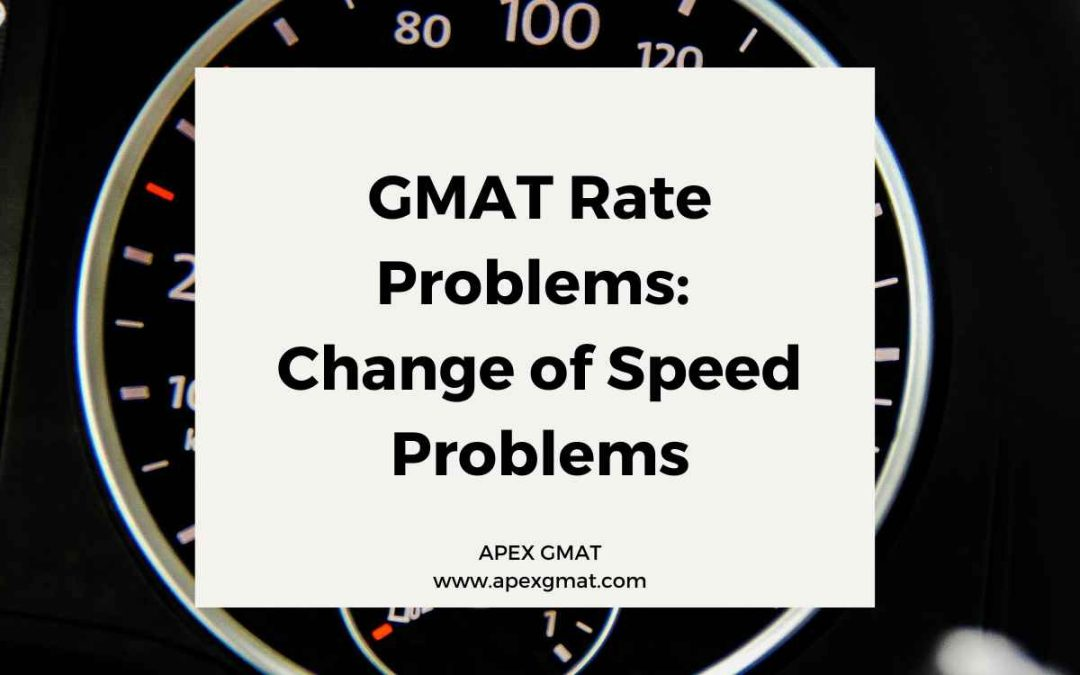## GMAT Rate Problems: Change of Speed Problems

Welcome back to our series on GMAT quant rate problems. In the last two articles, we saw how GMAT quant problems employ the concepts of average speed and instantaneous speed. This article will introduce methods for solving problems where the speed of a vehicle...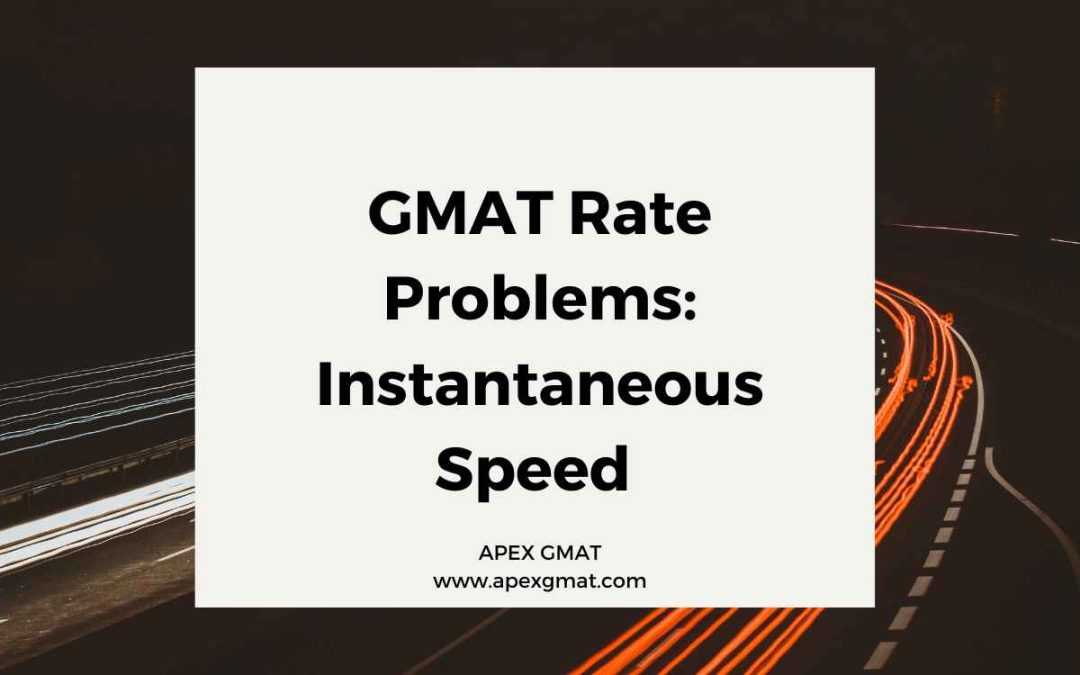## GMAT Rate Problems: Instantaneous Speed

Welcome back to our series on GMAT quant rate problems. In the last article, we learned about average speed and observed various ways that data sufficiency problems can try to “trick” test-takers with this concept. This article will contrast average speed against...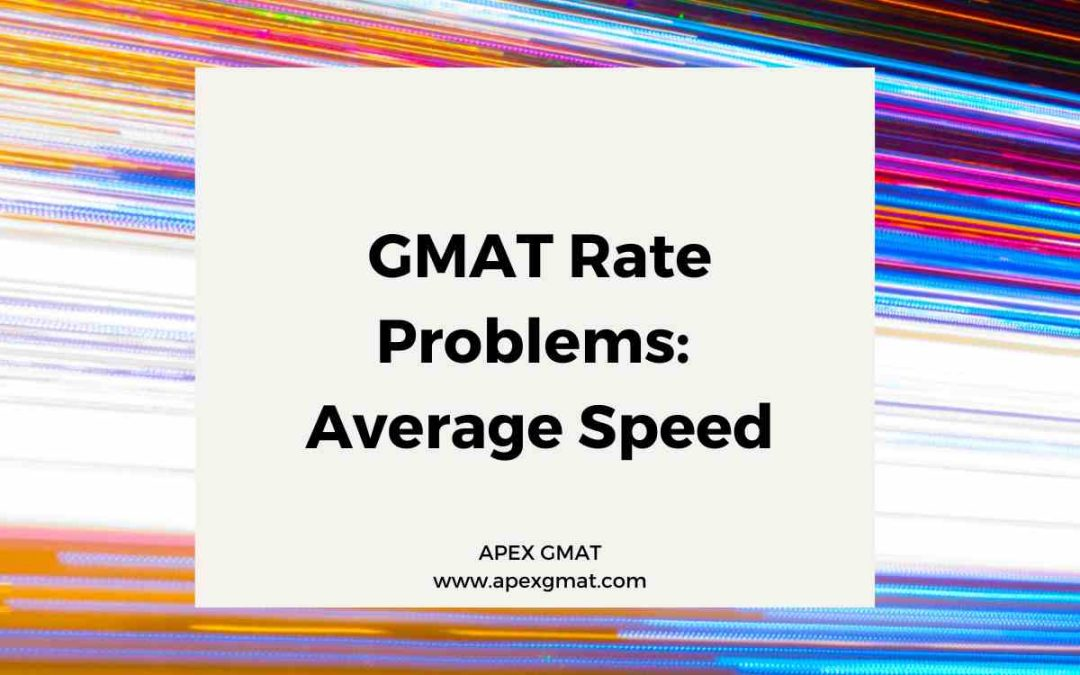## GMAT Rate Problems: Average Speed

Welcome back to our series on GMAT quant rate problems. This article and the next will introduce concepts related to average speed. Though average speed is a simple concept, there are many ways for GMAT quant problems to “trick” test-takers with average speed...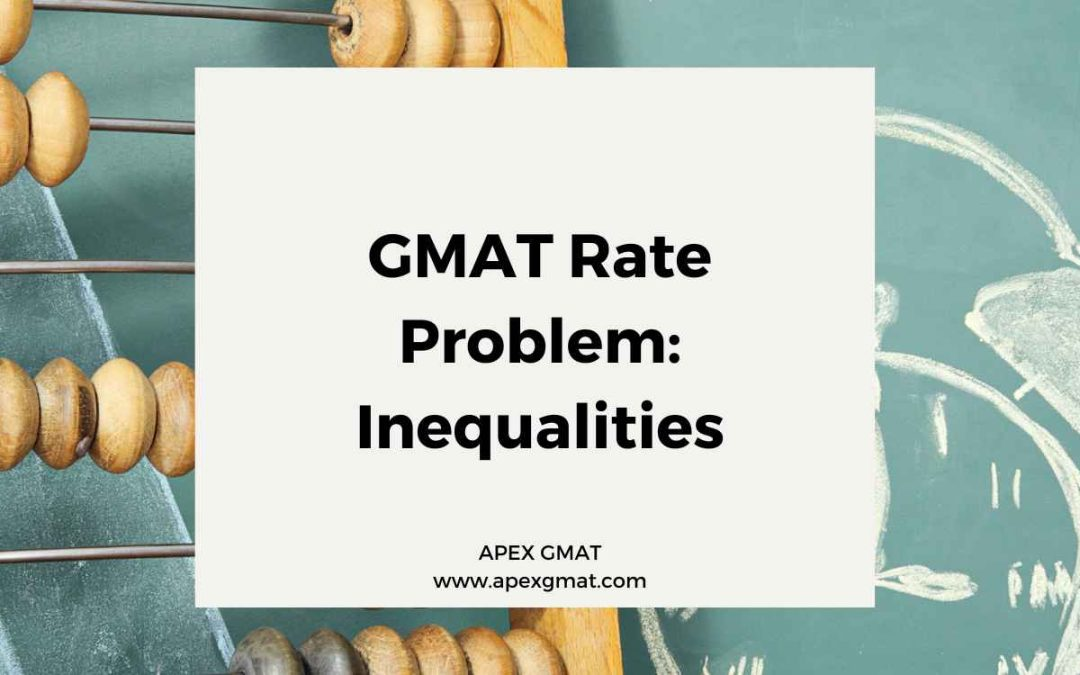## GMAT Rate Problems: Inequalities

Welcome back to our series on GMAT quant rate problems. The last article covered rate problems with geometry elements. In this article, we’ll see how rates are used in data sufficiency inequalities problems. Inequalities are a common form of data sufficiency problems,...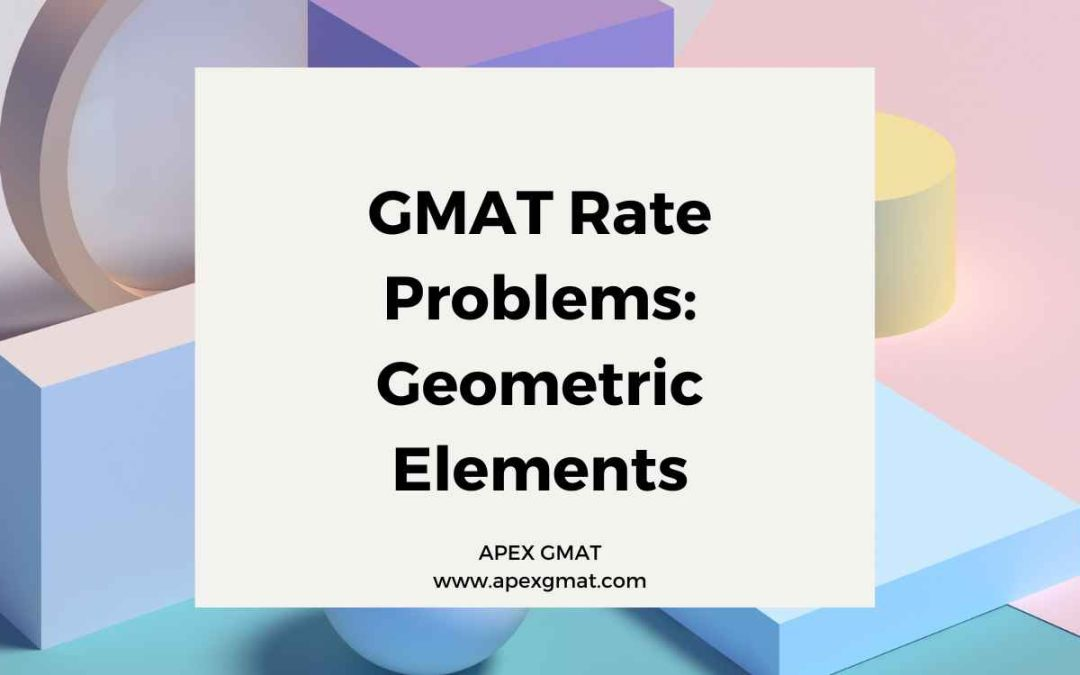## GMAT Rate Problems: Geometry

Welcome back to our series on GMAT quant rate problems. In the last article, we learned about problems involving fuel consumption rates. This article will address rates problems that incorporate geometry concepts. These are speed = distance/time problems where the...